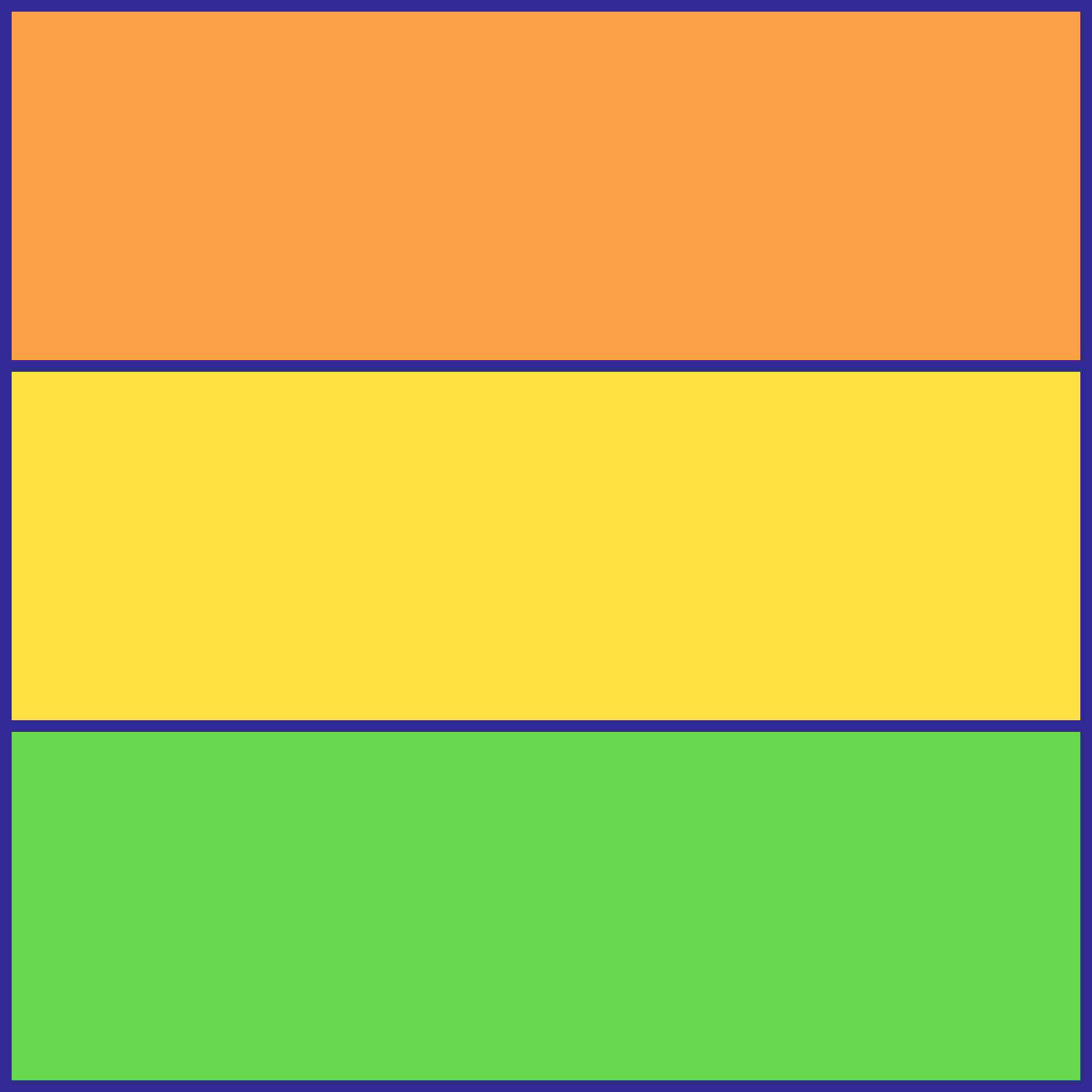# Square Trisection

A square is divided into three congruent rectangles. The area of one rectangle is 1200 units. What is the perimeter of the square?×